Courses

# Test: Nernst Equation

## 10 Questions MCQ Test Chemistry for JEE | Test: Nernst Equation

Description
This mock test of Test: Nernst Equation for JEE helps you for every JEE entrance exam. This contains 10 Multiple Choice Questions for JEE Test: Nernst Equation (mcq) to study with solutions a complete question bank. The solved questions answers in this Test: Nernst Equation quiz give you a good mix of easy questions and tough questions. JEE students definitely take this Test: Nernst Equation exercise for a better result in the exam. You can find other Test: Nernst Equation extra questions, long questions & short questions for JEE on EduRev as well by searching above.
QUESTION: 1

Solution:
QUESTION: 2

### Three cell A, B and C has equilibrium constant in the ratio 1:4 : 9 respectively. Arrange the following cells in the order of increasing Gibbs free energy.

Solution:

The correct answer is option A

The cells will be arranged as such 9 : 4: 1.

Explanation:

• The equilibrium constant that is used here is represented with k.

• The smaller the value of k the higher will be the value of energy according to Gibbs.

• So, 1 has the highest Gibbs free energy while 9 has the least.

• This is the energy of a chemical reaction.

• It is represented by G.

QUESTION: 3

### Gibbs free energy change for a cell reaction is positive what does it indicates?

Solution:

The correct answer is Option C.

Reactions with a negative ∆G release energy, which means that they can proceed without an energy input (are spontaneous). In contrast, reactions with a positive ∆G need an input of energy in order to take place (are non-spontaneous). Reactions with a positive ∆H and negative ∆S are non-spontaneous at all temperatures.

QUESTION: 4

At equilibrium:

Solution:

The correct answer is option A

E cell is 0 in equilibrium, that is Ecathode becomes equal to E anode ……….
EZero cell becomes zero when both the electrodes are of the same metal but of different concentration i.e for concentration cell……
We know that EZero cell is - Ezerocathode -E zeroanode (since cathode and anode are same ) so EZero =0

QUESTION: 5

In the equation, ΔG° = – nF E° cell ; F is:

Solution:

The correct answer is Option B.
The relationship between ΔGo and Eo is given by the following equation: ΔGo=−nFEo. Here, n is the number of moles of electrons and F is the Faraday constant.

QUESTION: 6

Consider the cell reaction:
Cd(s) | Cd2+ (1.0 M) || Cu2+ (1.0 m) | Cu (s)
If we wish to make a cell with more positive voltage using the same substances, we should:

Solution:

The correct answer is Option D.

Redox reaction:
Cd(s)→Cd2++2e
Cu2++2e→Cu(s)
Ecell = E°cell − (0.059/2) log ([Cd2+]/ [Cu2+])
Decreases [Cd2+] to 0.1M and increases [Cu2+] to 1.0M

QUESTION: 7

. The electrode potential at any concentration measured with respect to standard hydrogen electrode can be represented by:

Solution:

The correct answer is Option B
Nernst equation is,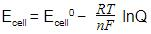where, Q is the reaction quotient of the reaction
As, [M (s) ]=1
We get,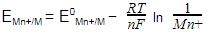QUESTION: 8

. For an equation: Ni(s) + 2Ag+(aq) → Ni2+ (aq) + 2Ag(s) the Nernst equation is written as:

Solution:

The correct answer is Option A.
Oxidation:
Ni(s) ---> Ni2+(aq) + 2e-
Reduction:
2Ni+(aq) + 2e- ----> 2Aq(s)
2e- are in the above reaction so;
n = 2
We know that
Ecell = Eocell – (RT/nF) lnKc
= Eocell – (RT/nF) ln ([Ni]2+ / [Ag+]2

QUESTION: 9

Nernst equation for an electrode is based on the variation of electrode potential of an electrode with:

Solution:

Nernst equation for an electrode is based on the variation of electrode potential of an electrode with temperature and concentration of electrolyte.

QUESTION: 10

The free energy change for the following cell reaction is given as :

2Au3+ (aq) + 3Cu (s) → 2Au (s) + 3Cu2+ (aq)

Solution:

The correct answer is Option D.

EO = EOCa2+/ Ca  -  EOAu2+/ Au
= -2.87 - (1.50)
= -2.87 - 1.50
= -4.37 V
rGO = nFEO
= -6 FEO

Track your progress, build streaks, highlight & save important lessons and more!

### Similar Content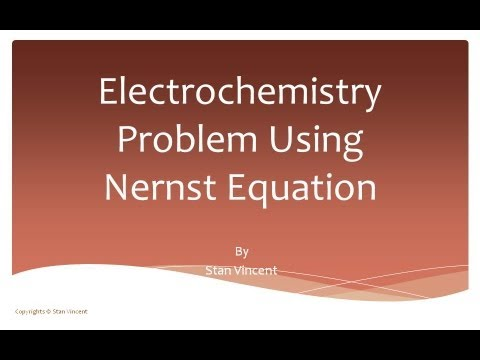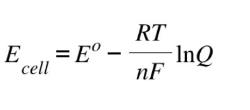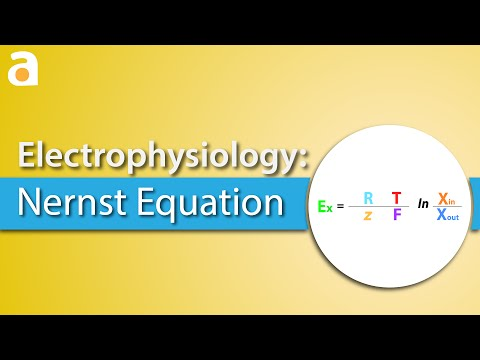### Related tests